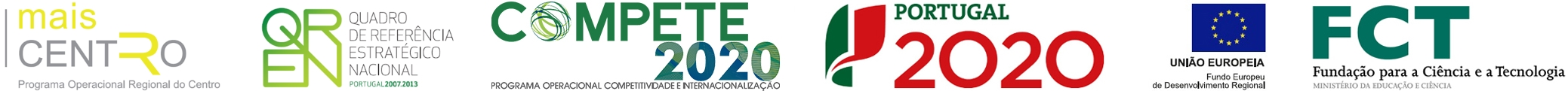Investigation of molecular interactions in binary mixture (benzyl benzoate plus ethyl acetate) at T = (308.15, 313.15, and 318.15) K: An insight from ultrasonic speed of sound and density
 authors Nayeem, SM; Nyamathulla, S; Khan, I; Rao, DK nationality International journal JOURNAL OF MOLECULAR LIQUIDS author keywords Speed of sound; Density; Excess properties; Partial molar volumes; Isentropic compressibility; Enthalpy; Entropy; Gibb's free energy keywords N-BUTYL ACETATE; BRANCHED-ALKANE MIXTURES; EXCESS MOLAR VOLUMES; LIQUID-MIXTURES; METHYL ACETATE; IONIC LIQUIDS; COSMO-RS; REFRACTIVE-INDEX; THERMODYNAMIC PROPERTIES; DIFFERENT TEMPERATURES abstract Speed of sound (u) and densities (rho) of binary liquid mixtures of benzyl benzoate with ethyl acetate, including pure liquids, over the entire composition range have been measured at T = (308.15, 313.15 and 318.15) Musing the experimental data such as excess molar volume (V-m(E)), excess acoustic impedance (Z(E)), excess intermolecular free length (L-f(E)), excess available volume (V-a(E)), excess surface tension (o(s)(E)), excess coefficient of thermal expansion (alpha(E)(P)), excess isotropic compressibility, (k(T)(E)), excess internal pressure (pi(E)(i)) and excess free volume (V-f(E)). Also enthalpy (H-E), entropy (S-E), Gibb's free energy (G(E)), partial molar volumes/partial molar compressibilities, excess partial molar volumes/excess partial molar compressibilities, partial and excess partial molar volume of the components at infinite dilution ((V) over bar (infinity)(m,1), (V) over bar (infinity)(m,2)), ((V) over bar (E infinity)(m,1) and (V) over bar (E infinity)(m,2)) and variations of H-E/S-E/G(E) with change in pressure at constant temperature have been evaluated. Molecular interactions in the system have been studied in the light of variation of excess values of calculated properties and these properties have been fitted to Redlich-Mister type polynomial equation. Further, Lennard-Jones potential repulsive exponent term (n) and relative association (R-A) are computed and interpreted to elucidate the molecular interactions in the liquid mixture. The present investigation also comprises evaluation of the acoustic non-linearity parameter (B/A) in the mixtures and calculation of cohesive energy Delta A, Van der Wall's constants (a, b) and distance of the closest approach (d). Moreover, speed of sound in the mixtures has been evaluated using various semi-empirical relations and such values were compared with experimental speeds to verify applicability to the systems investigated. The computational study allows a qualitative analysis of the results in terms of the individual contribution to the excess enthalpy and Gibb's energy in terms of electrostatic, van der Waals and hydrogen bonding interaction in the binary mixture. (C) 2016 Elsevier B.V. All rights reserved. publisher ELSEVIER SCIENCE BV issn 0167-7322 year published 2016 volume 218 beginning page 676 ending page 685 digital object identifier (doi) 10.1016/j.molliq.2016.02.045 web of science category Chemistry, Physical; Physics, Atomic, Molecular & Chemical subject category Chemistry; Physics unique article identifier WOS:000376800500077
ciceco authors
impact metrics
 journal analysis (jcr 2019): journal impact factor 5.065 5 year journal impact factor 4.766 category normalized journal impact factor percentile 81.277
 dimensions (citation analysis): altmetrics (social interaction):

Apoio